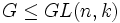# Any linear group is finite-dominating in the corresponding affine group over characteristic zero

## Statement

Suppose$k$ is a field of characteristic zero and$G$ is a subgroup of the general linear group$GL(n,k)$. Consider the group$A = k^n \rtimes G$, i.e., the affine group correpsonding to$G$. Then,$G$ is a Finite-dominating subgroup (?) in$A$: in other words, any finite subgroup of$A$ is conjugate in$A$ to a subgroup of$G$.

## Proof

Given: A group$G \le GL(n,k)$.$A$ is the semidirect product of$k^n$ and$G$. All the groups$G, A, GL(n,k)$ and$GA(n,k)$ are viewed here as subgroups of$GA(n,k)$.

1. By fact (1),$GL(n,k)$ is finite-dominating in$GA(n,k)$.
2. Since$A$ contains$k^n$, the product of$A$ and$GL(n,k)$ is$GA(n,k)$. Also, the intersection of$GL(n,k)$ with the group$A$ is$G$. Thus, by fact (2),$G$ is finite-dominating in$A$.